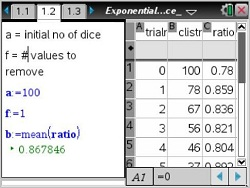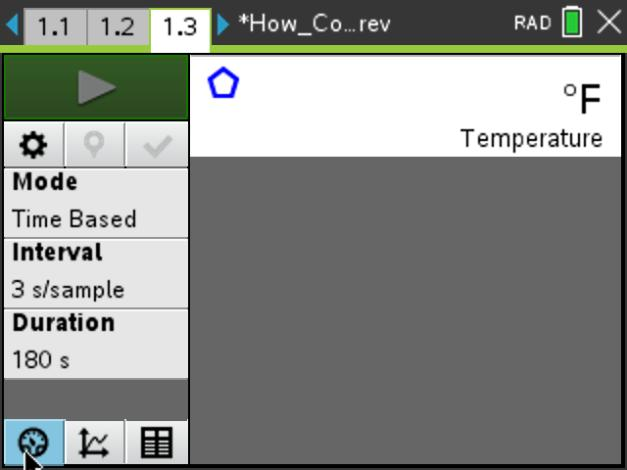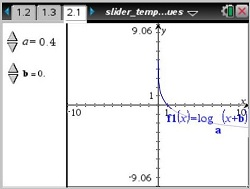### Comparing Exponential and Power Functions

Students will be able to use various graphical representations to determine which of two functions is greater for large values of x.
•TI-Nspire™ CX/CX II
•TI-Nspire™ CX CAS/CX II CAS
• TI-Nspire™ Navigator™
• TI-Nspire™
• TI-Nspire™ CAS### Compound Interest

This lesson involves exploring the formula for compound interest as a function of the initial deposit, interest rate, and the number of pay periods per year.
•TI-Nspire™ CX/CX II
•TI-Nspire™ CX CAS/CX II CAS
• TI-Nspire™ Navigator™
• TI-Nspire™
• TI-Nspire™ CAS### Exponential Dice

This lesson involves using a simulation to generate data that can be modeled by exponential growth and decay functions.
•TI-Nspire™ CX/CX II
•TI-Nspire™ CX CAS/CX II CAS
• TI-Nspire™ Navigator™
• TI-Nspire™
• TI-Nspire™ CAS### How Cool It Is

This lesson involves creating an exponential regression equation to model the temperature of water as it cools.
•TI-Nspire™ CX/CX II
•TI-Nspire™ CX CAS/CX II CAS
• TI-Nspire™ Navigator™### Logarithmic Transformations of Data

This lesson involves three real-world data sets in which the relationship between each pair of variables is non-linear. Students will be asked to describe the original relationship between each pair of variables, and observe how each transformation is used to achieve a linear relationship.
•TI-Nspire™ CX/CX II
•TI-Nspire™ CX CAS/CX II CAS
• TI-Nspire™ Navigator™
• TI-Nspire™
• TI-Nspire™ CAS### Slider Template Using Specific Values

In this activity, students will learn how to create a slider to control a variable on a graph.
•TI-Nspire™ CX/CX II
•TI-Nspire™ CX CAS/CX II CAS
• TI-Nspire™ Navigator™
• TI-Nspire™
• TI-Nspire™ CAS### Transformations of Exponential Functions

Students will explore the family of exponential functions of the form f(x) = c * b x+aand be able to describe the effect of each parameter on the graph of y = f(x).
•TI-Nspire™ CX/CX II
•TI-Nspire™ CX CAS/CX II CAS
• TI-Nspire™ Navigator™••